# How to Convert Exponential Large Number to Normal Integer in Excel?

• Last Updated : 02 Jun, 2022

Excel is powerful data visualization and data management tool which can be used to store, analyze, and create reports on large data. It is generally used by accounting professionals for the analysis of financial data but can be used by anyone to manage large data. Data is entered into the rows and columns.

### Converting Exponential Large Number to Normal Integer in Excel

Excel automatically converts the number into scientific notation if you enter a number having a length of more than 11 digits. For example: If you enter the value -> 123456789002, it will get converted to ‘1.23457E + 11’. Scientific notation is hard to understand so it should be converted to normal use for further use or analysis.

There are various methods to perform the above task,

1. Adding a single quote before.
2. Using format cell functions.
3. Using the formula

Method 1: Using Single Quote

This is the simplest way. It prevents the numbers to get converted into scientific notation. We just have to add a single quote(‘) before the value while entering the value (more than an 11-digit number).

Input: Adding a single quote before the value.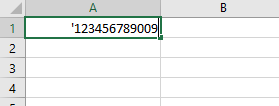Output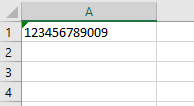But adding the quote before every value can be a difficult task if we need to enter a large amount of data.

Method 2: Using the format cell option

This method can be used to convert many values at a time. Suppose we have many scientific values as given below: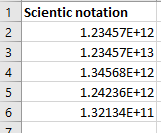Follow the below steps to convert it into normal values

Step 1: Select the data you want to convert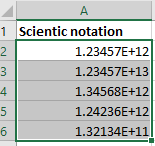Step 2: Right-click and select the ‘Format Cells’ options.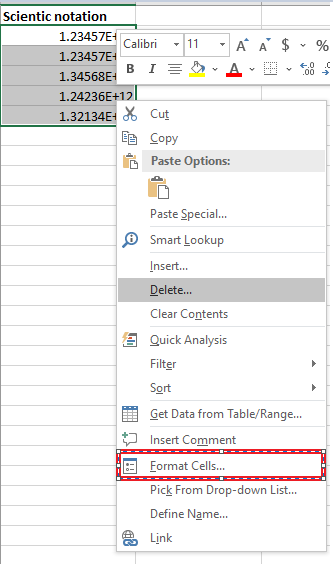Step 3: Under the Number tab, click Custom from the Category list box and input the number 0 into the Type box.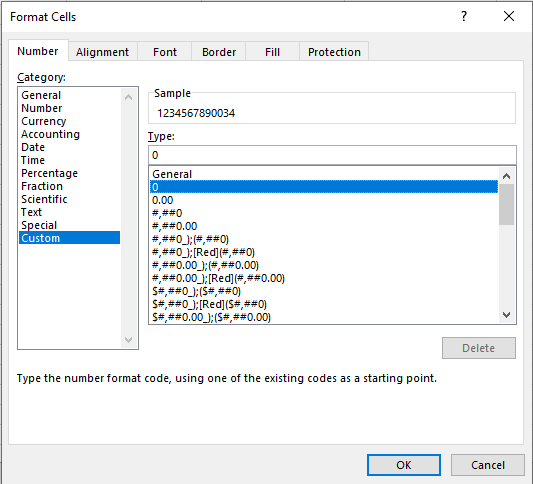Step 4: Select OK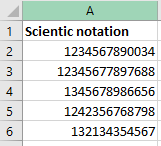Method 3: Using the formula

TRIM (Cell_name): this formula will convert the scientific notation to a normal integer.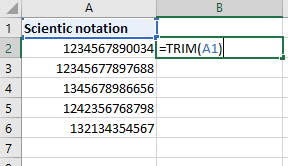Drag the fill handle over to the data range.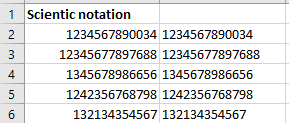You can see that all the notation is converted to a normal integer value. This method can be used if you want the result in a cell other than the one in which scientific notation is present.

My Personal Notes arrow_drop_up
Recommended Articles
Page :